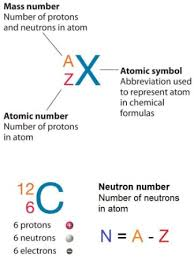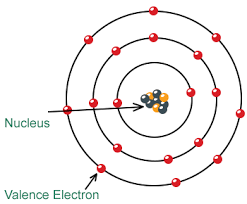# What are The Neutron Symbol Mass and Charge

Hello friends, last time we discussed the charge of a neutron. Today we will talk about the mass and charge of neutrons and how you can find the number of electrons in an atom.

Generally, the atomic structure consists of a positively charged nucleus (consisting of protons and neutrons) holding together the negatively charged electrons in the orbitals with a strong binding force.

### What is the Correct Symbol for a NeutronSince neutron is a subatomic particle with no electric charge, its symbol is n or n0. To use a more accurate symbol, the nuclear symbol for a neutron is 0n1, which again indicates that they are neutral or charge fewer particles and have slightly higher mass as compared to protons.

How to Find the Number of ElectronsAn electron is the smallest and the most reactive sub-atomic particles, which revolve around their orbitals. As a chemistry student, you would have required to find out the number of the electron for various reasons. Here, we provide you with three simple steps to find out the number of electrons of an atom.
1. Take a Periodic table and find the atomic mass and an atomic number of the particular element.
Atomic number is equivalent to the number of protons and atomic mass is equivalent to the sum of a number of protons and neutrons.
2. Then subtract the atomic mass from the atomic number.
3. The resultant number you get is the number of electrons in the atom.

### Mass of Protons Neutrons and Electrons

Following are the masses of Protons, Neutrons and Electrons :

Protons: 1.6726219 × 10-27 kilograms. This is called one atomic mass unit (or amu)

Neutrons: The mass of neutron cannot be determined due to its zero (or neutral) charge. But it is slightly greater than protons.

Electrons: The mass of electrons is approximately 9.109×1031 kilograms.